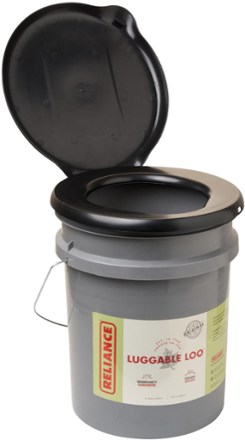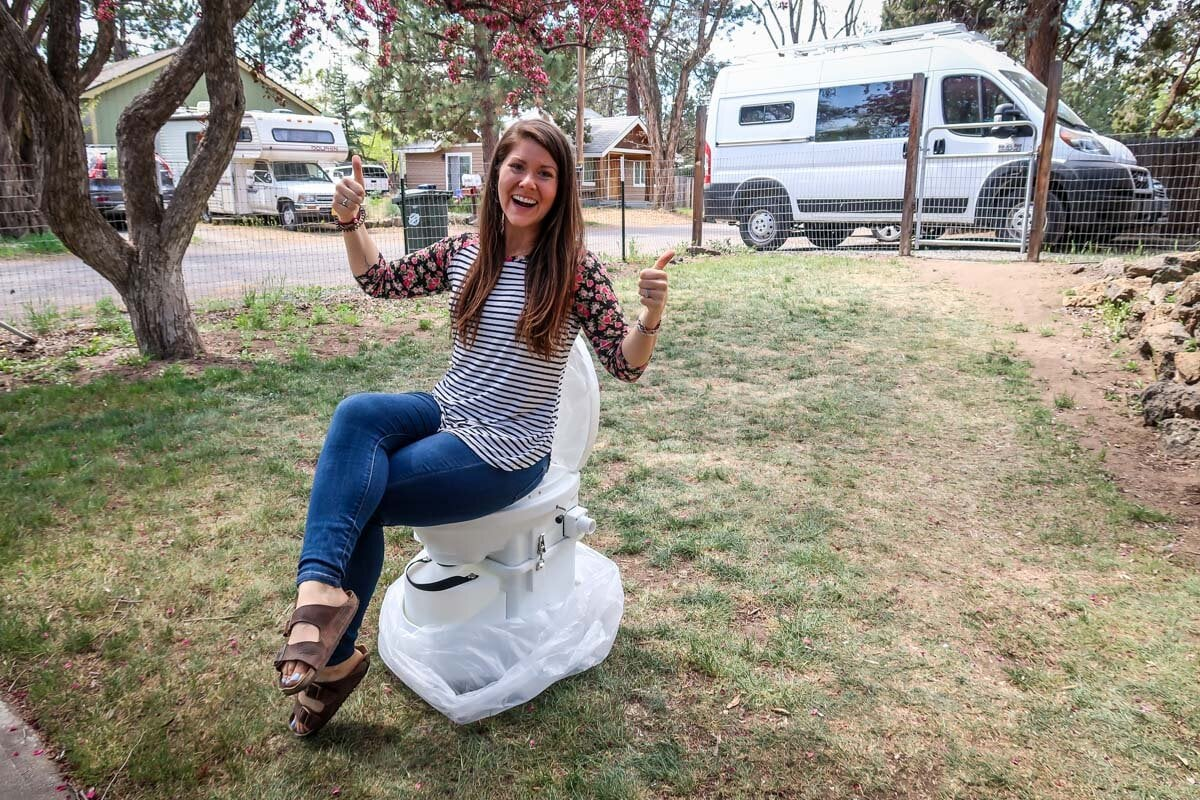## 二十笔实用成语 1175

royl

### 二十笔实用成语 1175

01. where the rubber hits/meets the road

02. where the shoe pinches

03. where there is a will, there is a way

04. where there's life there's hope

05. where there's muck there's brass06. where there's smoke, there's fire

07. where to?

09. Where would we be then?
[成语句型]我们届时将在哪里? 我们届时将如何自处?

11. where'd you get that?

12. where's John when we need him?

13. where's the beef?

14. where's the fire?

15. where's the harm?

17. wherever that is

18. wherever that may be

19. wherever you are

20. whet one's appetite, to